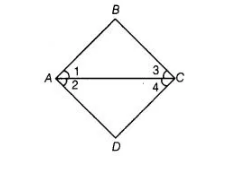`
Question:

In the adjoining figure, we have ∠1 =∠3 and ∠2 = ∠4. Show that ∠A = ∠C.Solution:

Given,∠1 = ∠3 …(i)

and ∠2 = ∠4 …(ii)

According to Euclid’s axiom, if equals are added to equals, then wholes are also equal.

On adding Eqs. (i) and (ii), we get

∠1 + ∠2 = ∠3 +∠4

=> ∠A = ∠C